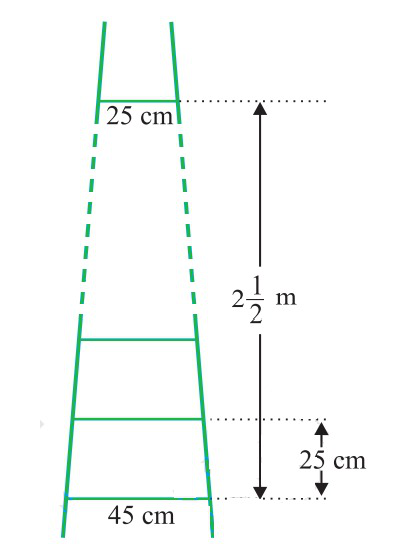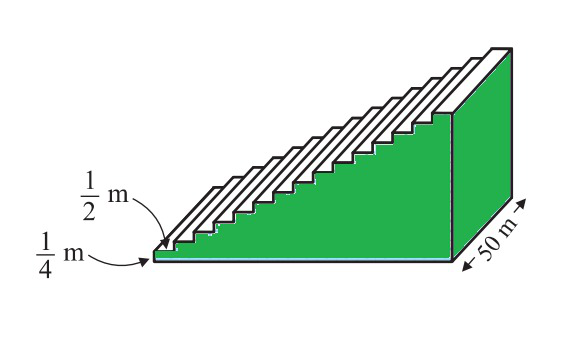Related Articles
Class 10 NCERT Solutions- Chapter 5 Arithmetic Progressions – Exercise 5.4
• Last Updated : 21 Feb, 2021

### [Hint : Find n for an < 0]

Solution:

Given the AP series is 121, 117, 113, . . .,

Here, first term, a = 121 and Common difference, d = 117-121= -4

nth term formula, an = a+(n −1)d

Hence,

an = 121+(n−1)(-4)

= 121-4n+4

=125-4n

To find the first negative term of the series, an < 0 Therefore,

125-4n < 0

125 < 4n

n>125/4

n>31.25

Therefore, the first negative term of the series is 32nd term (n=32).

which will be, a32 =125-4(32) = -3

### Question 2. The sum of the third and the seventh terms of an AP is 6 and their product is 8. Find the sum of first sixteen terms of the AP.

Solution:

nth term formula, an = a+(n −1)d

Third term, a3 = a+(3 -1)d

a3 = a + 2d

And Seventh term, a7= a+(7-1)d

a7 = a + 6d

According to given conditions,

a3 + a7 = 6 …………………………….(i)

a3 × a7 = 8 ……………………………..(ii)

Substituting the values in eqn. (i), we get,

a+2d +a+6d = 6

2a+8d = 6

a+4d=3

a = 3–4d …………………………………(iii)

Now substituting the values in eqn. (ii), we get,

(a+2d)×(a+6d) = 8…………………..(iv)

Putting the value of a from eqn. (iii) in eqn. (iv), we get,

(3–4d +2d)×(3–4d+6d) = 8

(3 –2d)×(3+2d) = 8

32 – (2d)2 = 8         (using the identity, (a+b)(a-b) = a2-b2)

9 – 4d2 = 8

4d2 = 9-8 = 1

d = √(1/4)

d = ±1/2

d = 1/2 or -1/2

So now, if

d = 1/2,

a = 3 – 4d = 3 – 4(1/2) = 3 – 2 = 1

and if d = -1/2,

a = 3 – 4d = 3 – 4(-1/2) = 3+2 = 5

Sum of nth term of AP is: Sn = n/2 [2a +(n – 1)d]

So, when a = 1 and d=1/2

Then, the sum of first 16 terms are;

S16 = 16/2 [2 +(16-1)1/2] = 8(2+15/2)

S16 = 76

And when a = 5 and d= -1/2

Then, the sum of first 16 terms are;

S16 = 16/2 [2.5+(16-1)(-1/2)] = 8(5/2)

S16 = 20

### [Hint : Number of rungs = 250/25 + 1]Solution:

As it is given that,

Distance between the rungs of the ladder is 25 cm.

Distance between the top rung and bottom rung of the ladder will be (in cm) = 2 ½ × 100 = 250 cm

Hence, the total number of rungs = 250/25 + 1 = 11

As we can observe here, that, the ladder has rungs in decreasing order from top to bottom. Thus, the rungs are decreasing in an order of AP.

So, According to given condition

First term, a = 45

Last term, l = 25

Number of terms, n = 11

And the length of the wood required for the rungs will be equal to the sum of the terms of AP series formed.

Now, as we know, sum of nth terms is equal to, Sn= (n/2)(a+ l)

Sn= 11/2(45+25) = 11/2(70) = 385 cm

Hence, the length of the wood required for the rungs is 385cm.

### [Hint : Sx – 1 = S49 – Sx]

Solution:

According to the given statement,

Row houses are numbered in a row that are in the form of AP, which is as follows:

Here, according to the given condition,

First term, a = 1

Common difference, d=1

and last term = l

Let us say the number of xth houses can be represented as: Sx – 1 = S49 – Sx

Where Sx represents to sum of xth houses.

(Value of x such that the sum of the numbers of the houses preceding the house numbered x is equal to the sum of the numbers of the houses following it)

As, Sum of nth term of AP, Sn= n/2[a+l]

Sum of number of houses beyond x house = Sx-1

Sx-1 = (x-1)/2[1+(x-1)]

Sx-1 = x(x-1)/2 ………………………………………(i)

By the given condition, we can write,

S49 – Sx = {49/2[1+(49)}–{x/2(1+x)}

= 25(49) – x(x + 1)/2 ………………………………….(ii)

As eqn. (i) and (ii) are equal, So

x(x-1)/2 = 25(49) – x(x-1)/2

x(x-1) = 25(49)

x = ±35

As we know, the number of houses cannot be a negative number.

Hence, the value of x is 35.

### [Hint : Volume of concrete required to build the first step = 1/4 × 1/2 × 50 m3]Solution:

As we can see from the given figure,

1st step is ¼ m high, ½ m wide and 50 m long

2nd step is (¼+¼ = ½ m) high, ½ m wide and 50 m long and,

3rd step is (3×¼ = 3/4 m) high, ½ m wide and 50 m long.

and so on

Hence, we can conclude that the height of step increases by ¼ m each time when width and length is ½ m and 50 m respectively.

So, the height of steps forms a series AP in such a way that;

¼, ½ , ¾, 1, 5/4, ……..

Volume of steps = Volume of Cuboids

= Length × Breadth × Height

Now,

Volume of concrete required to build the first step = ¼ ×½ ×50 = 25/4

Volume of concrete required to build the second step =½ ×½×50 = 25/2

Volume of concrete required to build the second step = ¾ × ½ ×50 = 75/2

Volume of steps = ½ × 50 × (¼ + 2/4 + 3/4 + 4/4 + 5/4 + …….) …………………….(1)

Now, we can see the height of concrete required to build the steps, are in AP series;

Thus, applying the AP series concept to the height,

First term, a = 1/4

Common difference, d = 2/4-1/4 = 1/4

n = 15

As, the sum of n terms is : Sn = n/2[2a+(n-1)d]

Sn = 15/2(2×(1/4 )+(15 -1)1/4)

Sn = 15/2 (4)

Sn = 30

Hence, up solving eqn. (1), we get

Volume of steps = ½ × 50 × Sn

= ½ × 50 × 30

= 750 m3

Hence, the total volume of concrete required to build the terrace is 750 m3.

Attention reader! Don’t stop learning now. Get hold of all the important DSA concepts with the DSA Self Paced Course at a student-friendly price and become industry ready.

My Personal Notes arrow_drop_up